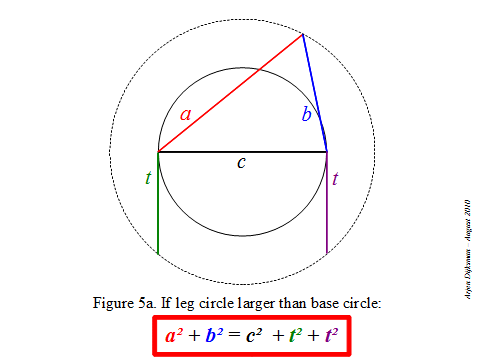## Saturday, August 28, 2010

### Alternative Pythagorean quadruples and other extensions to Pythagoras theorem

The vertices of an arbitrary triangle can be disposed onto two concentric circles such that the base is the diameter of the first circle (which I call the base circle) and the opposite vertex is on the second circle (which I call the leg circle). As there are three bases, there are generally three ways to arrange this setting. I mentioned in my preceding post that we can apply the Pythagorean-like relation a²+b²=c²±2t² to this triangle, where c is its base, a and b are the legs and t is the tangent to the inner circle emanating from the outer circle. For clarity, I reproduce an illustrative figure from my preceding post, for the case where the inner circle is the base circle.Now, like for the classical Pythagoras relation, there are many features that can be investigated regarding this alternative relation. For example, I would like to better understand the relation of this 2D formula with the Cartesian 3D variant of Pythagoras: x²+y²+z²=d², which is analogous to the case where the leg circle is smaller than the base circle (see Figure 5c of preceding post). Another interesting aspect is the fact that the square of the tangent (multiplied by π) determines the area of the annular ring delimited by the base and the leg circles, as stated by Mamikon Mnatsakanian. This fact offers possibilities for areal representations of the squares or circles related to the sides of the arbitrary triangle, like we are acquainted to do with the squares related to the sides of a right triangle. Also, what would be interesting to develop is its relation with the law of cosines (as noticed by Pat Ballew) and the angle subtended by sides a and b. I guess this will leave enough stuff for future posts or conversations, or even papers in specialized journals.

As a conclusion, let me mention Pythagorean numerological features which provide stuff for entertaining puzzles. Maybe you know the Pythagorean triples, those sets of integer numbers (n,m,l) that verify n²+m²=l² and of which (3,4,5) is the simplest instance. The 3D Pythagoras relation allows an extension to Pythagorean quadruples, sets of integer numbers (n,m,l,k) that satisfy n²+m²+l²=k² and of which (1,2,2,3) is the simplest instance. I couldn't resist to look for some integer quadruples that satisfy a²+b²=c²+2t². Among them I found two nice quadruples with successive a,b,c :

(7,8,9,4) for which 7² + 8² = 9² + 2 × 4² = 113

(35,36,37,24) for which 35² + 36² = 37² + 2 × 24² = 2521